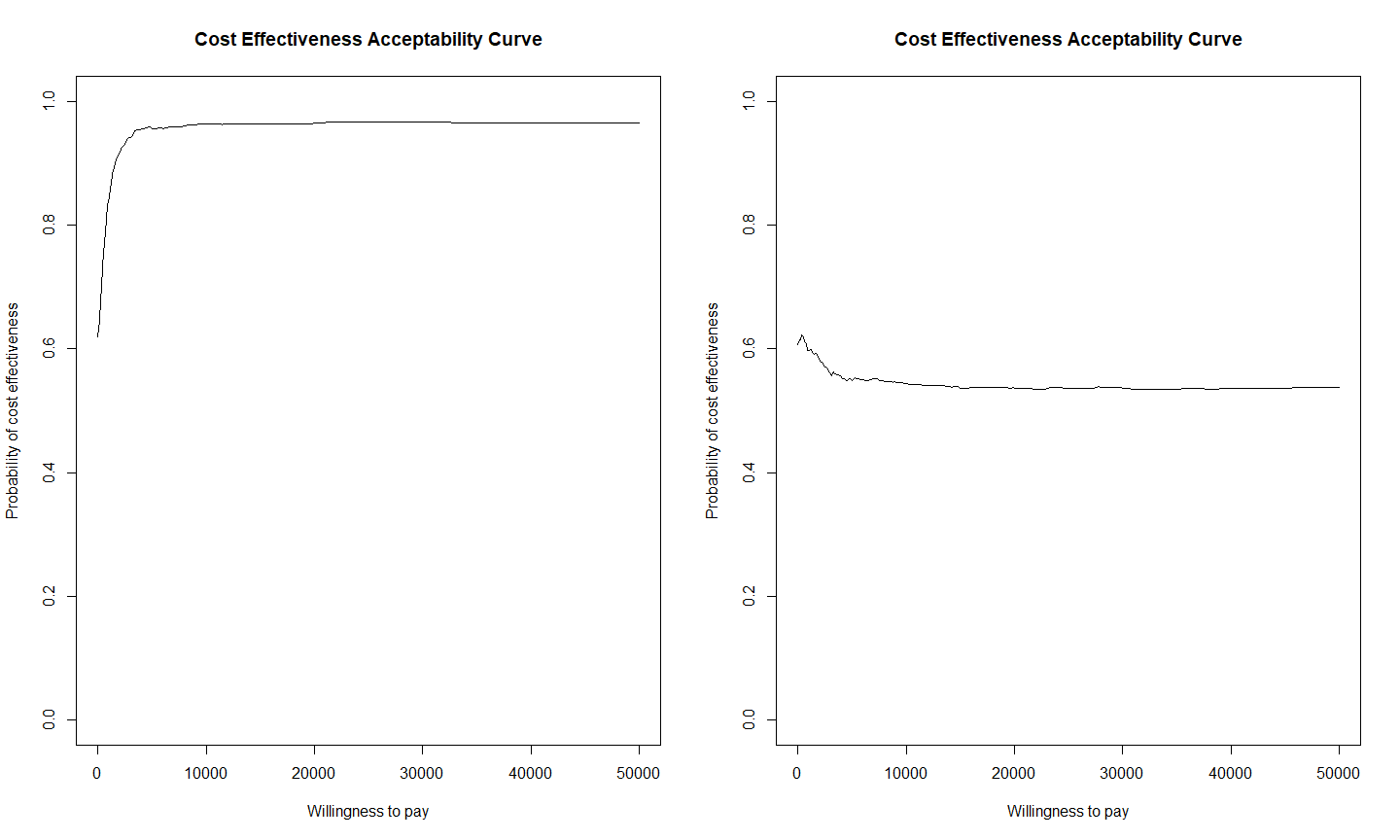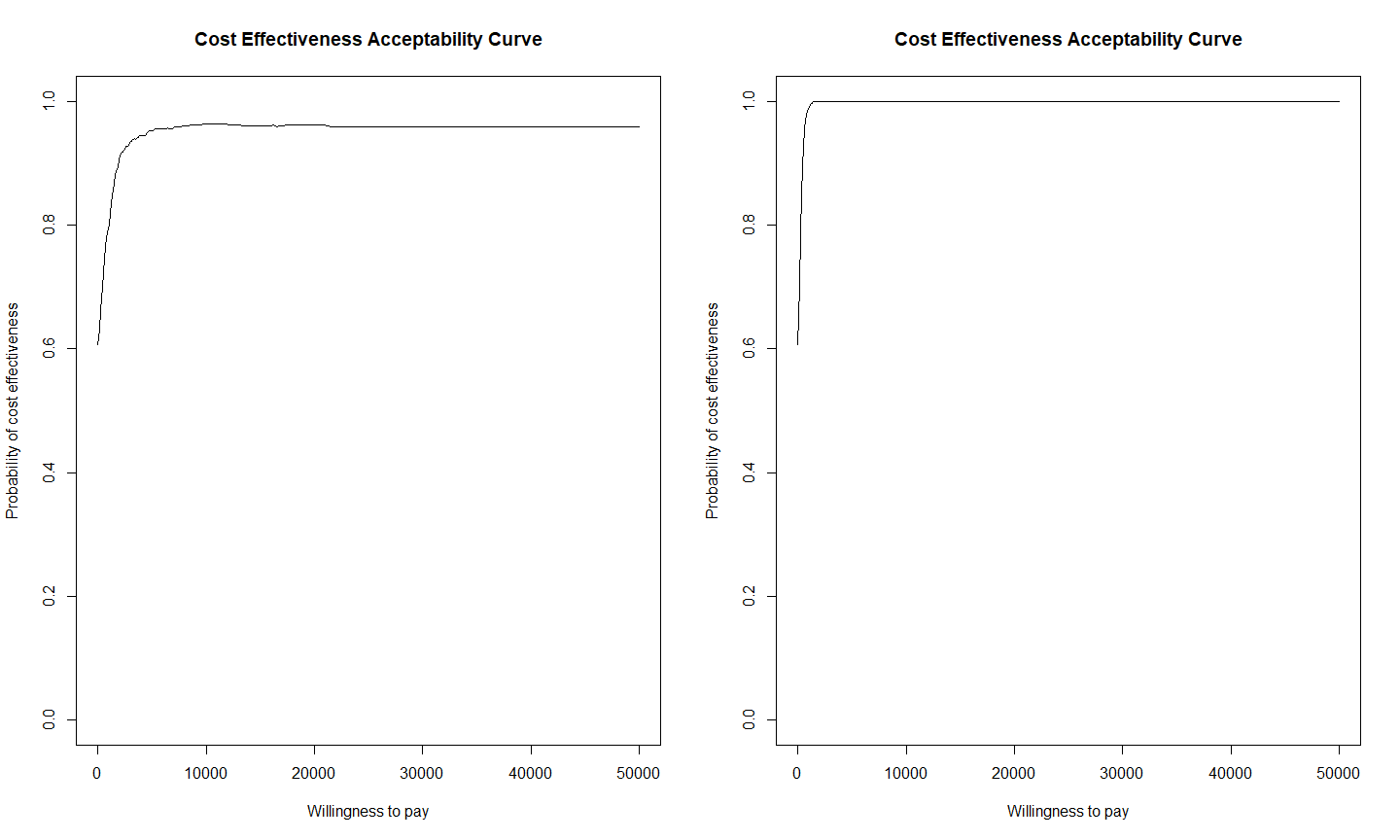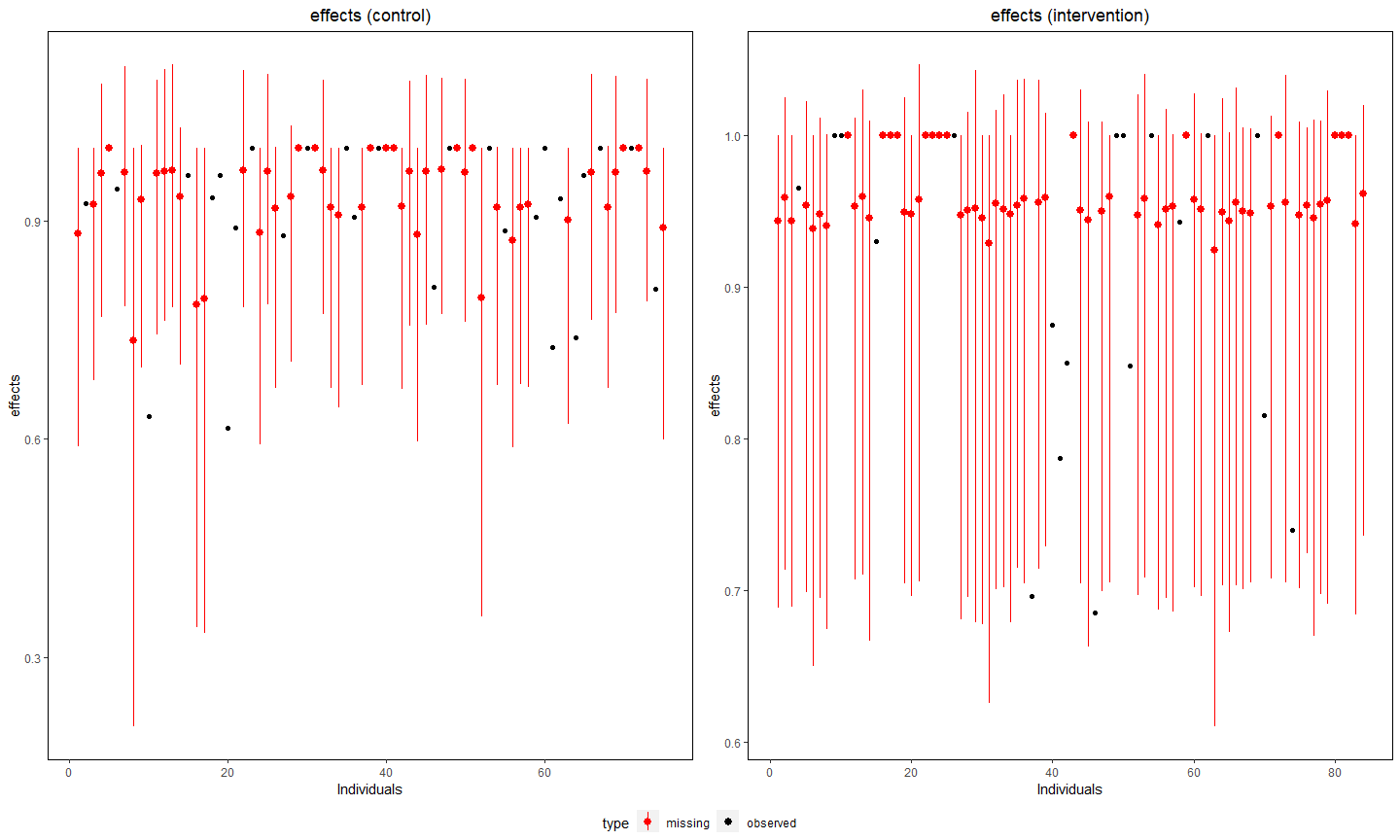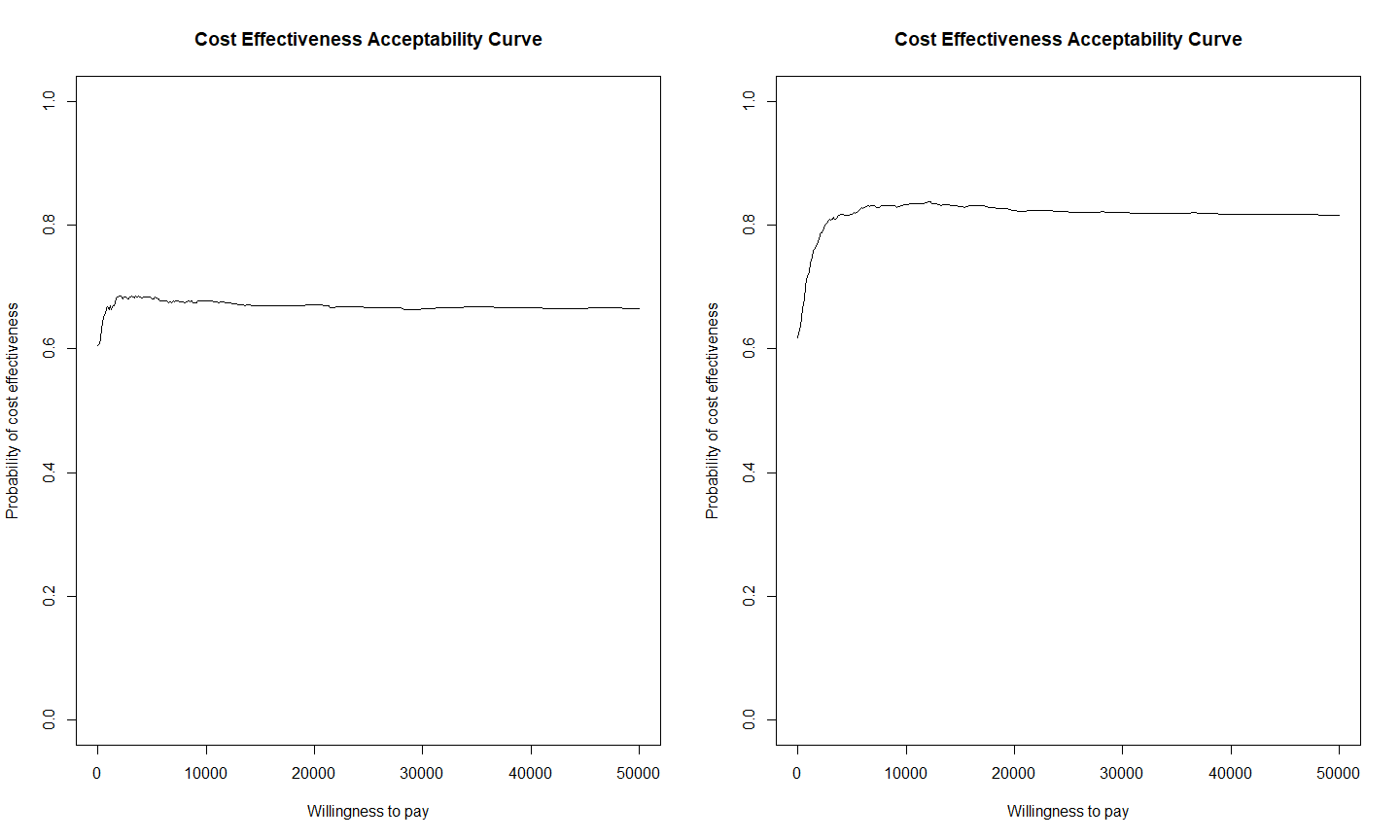# Fitting MNAR models in missingHE

When analysing partially-observed data, any statistical method makes either explicit or implicit assumptions about the missing values which can never be verified from the data at hand. Typically, most analyses rely on a missing at random (MAR) assumption, that is they assume that the observed data are able to fully explain missingness. However, there is always the chance that this assumption is not correct and missingness may depend on some values which are not observed, leading to a missing not at random (MNAR) assumption. Thus, it is extremely important that the robustness of the results to a range of alternative missingness assumptions is assessed in sensitivity analysis, including MNAR.

Each of the three types of missingness models in missingHE, namely selection, pattern mixture, and hurdle models, can be fitted under MNAR for either or both the effectiveness and cost outcomes. In missingHE, MNAR assumptions are codified in terms of some suitably-defined departures from MAR for the mean effectiveness and cost parameters, which are the main quantities of interest in the economic evaluation. This tutorial shows how MNAR assumptions can be specified for each type of model in missingHE. Throughout, we will use the built-in dataset called MenSS as a toy example, which is directly available when installing and loading missingHE in your R workspace. See the vignette called Introduction to missingHE for an introductory tutorial of each function in missingHE and a general presentation of the data from the MenSS dataset. See also the vignette called Model customisation in missingHE for few examples on how to customise the functions in missingHE to handle different types of issues in the analysis.

If you would like to have more information on the package, or would like to point out potential issues in the current version, feel free to contact the maintainer at [email protected]. Suggestions on how to improve the package are also very welcome.

## Handling MNAR with selection models

Selection models specify MNAR assumptions by directly modelling the missingness mechanisms, that is the models for the missing data indicators, as a function of some partially-observed variables. In missingHE, MNAR is defined by including the outcome variables ($$e$$ and $$c$$) inside the logistic regression models for the corresponding missing indicators (model.me and model.mc). Since some outcome values are not observed, the parameters capturing the dependence between missingness and the outcomes (called delta.e and delta.c) cannot be fully estimated from the observed data but are, at least partially, informed from some external sources of information, therefore defining a MNAR assumption. Two sources of external information are used to identify these parameters: informative prior distributions and distributional assumptions.

Informative priors on delta.e and delta.c can be provided in the form of a list object containing the hyperprior values to be passed to the selection function using the optional argument prior. By default, missingHE specifies standard normal distributions on these parameters, which are likely to have little impact compared to the results under MAR (i.e. when the parameters are set to zero). Different values for the priors on delta.e and delta.c can be passed to the function to overwrite the default values to assess the robustness of the results to different choices. The type of distributions for each outcome can be specified among a pre-defined set of choices using the arguments dist_e and dist_c. Type help(selection) to access the full list of available distributions for $$e$$ and $$c$$.

For example, we can fit a selection model under MNAR assumptions for the effectiveness variables (QALYs) in the MenSS dataset using the selection function. We must set the argument type = "MNAR" and then add the terms e inside the model for the corresponding missingness indicator, namely model.me = me ~ e.

> NN.sel1=selection(data = MenSS, model.eff = e ~ u.0, model.cost = c ~ 1,
+   model.me = me ~  e, model.mc = mc ~ 1, type = "MNAR",
+   n.iter = 1000, dist_e = "norm", dist_c = "norm")

The model assumes normal distributions for both outcomes and includes the baseline utilities as covariates in the model of $$e$$. Since we did not provide any prior, default prior values are used for the MNAR parameter delta.e. missingHE allows a flexible specification in terms of the variables assumed to be MNAR (either $$e$$, $$c$$ or both). In addition, other fully-observed variables can be included into the models model.me and model.mc, either under MAR or MNAR, to improve the estimation of the missingness probabilities. We can retrieve the estimates for the mean effectiveness and cost outcomes from the model using the print function.

> print(NN.sel1)
mean     sd    2.5%   97.5%  Rhat n.eff
mu_c 209.956 52.249 111.556 312.403 1.012   580
mu_c 189.938 39.945 113.229 264.546 1.013   170
mu_e   0.872  0.016   0.839   0.904 1.009   210
mu_e   0.922  0.022   0.883   0.964 1.008   200

We now consider an alternative MNAR specification where we provide some informative prior distributions on delta.e. In general, it is difficult to attach any specific interpretation to the values for this parameter because its effect may vary depending on the type of distributional assumptions made. We first define our prior values by creating a list object called my.prior. Within this list, we create a vector of length two called "delta.prior.e" which contains the prior values to be passed to selection.

> my.prior <- list(
+   "delta.prior.e" = c(10, 1)
+ )

As a simple exercise, we increase the prior mean of delta.e to $$10$$ to assess the impact on posterior estimates of a more informative prior about this parameter (relatively high positive value on the logit scale). We then fit the second MNAR model using the new prior values by setting the argument prior = my.prior

> NN.sel2=selection(data = MenSS, model.eff = e ~ u.0, model.cost = c ~ 1,
+   model.me = me ~  e, model.mc = mc ~ 1, type = "MNAR",
+   n.iter = 1000, dist_e = "norm", dist_c = "norm", prior = my.prior)

We can now check the results and compare them to those obtained under the first MNAR model.

> print(NN.sel2)
mean     sd    2.5%   97.5%  Rhat n.eff
mu_c 208.152 52.202 108.603 307.084 1.001  1000
mu_c 190.555 38.189 115.044 267.196 1.000  1000
mu_e   0.974  0.036   0.913   1.055 1.000  1000
mu_e   0.979  0.034   0.930   1.053 1.012  1000

We see that, with respect to the results from NN.sel1, the mean effectiveness estimates are on average higher in both treatment groups. To have a better idea of the impact in terms of cost-effectiveness conclusions for the different MNAR assumptions, we can use the function ceac.plot inside the package BCEA to display the cost-effectiveness acceptability curves based on the results from each model.

> par(mfrow=c(1,2))
> BCEA::ceac.plot(NN.sel1$cea) > BCEA::ceac.plot(NN.sel2$cea)The comparison between the two graphs shows that CEA conclusions are substantially affected by the specific assumptions made about the missing effects, therefore suggesting that the results of the model are not robust to the missingness assumptions considered. It is very important that the specific MNAR scenarios explored are informed based on some external information (e.g. expert opinion) so to provide a range of plausible assumptions to assess.

## Handling MNAR with pattern mixture models

Pattern mixture models specify MNAR assumptions through the combinations of two elements: identifying restrictions and sensitivity parameters. Since these models are defined within each missingness pattern, parameters that cannot be idenfitied from the patterns are typically identified by imposing some modelling restrictions, that is they are set equal to the corresponding parameters from other patterns which can be identified from the observed data.

For example, the complete case restriction identifies all unidentified parameters in each pattern by setting them equal to those estimated from the complete cases. Under MAR, these restrictions are the only element used to achieve the identification of the model. However, when MNAR assumptions are specified, identifying restrictions are combined with sensitivity parameters, that is parameters that are entirely identified based on evidence external to the data, to achieve the identification of the model. Sensitivity parameters are identified based on informative prior distributions but, unlike the priors for the MNAR parameters in selection models, they have more natural interpretations in terms of the impact on the posterior results.

missingHE allows the specification of MNAR assumptions for either or both outcome variables using the function pattern via the arguments restriction, Delta_e and Delta_c. The first is the type of identifying restrictions imposed: available choices are complete case ("CC") or available case ("AC") restrictions. The second and third are the prior values for the sensitivity parameters associated with the mean effectiveness and costs. Under MAR, these are set to $$0$$ (default values). Under MNAR, prior values for these parameters must be provided by the user in the form of a $$2\times2$$ matrix. For example, assuming a MNAR mechanism for the effectiveness, a possible choice for the prior values on Delta_e is

> Delta_e <- matrix(NA, 2, 2)
> Delta_e[1, ] <- c(- 0.3, - 0.2)
> Delta_e[2, ] <- c(-0.1, 0)

The rows represent the treatment group while the columns represent the lower and upper bounds for the uniform prior distributions that missingHE assumes for these parameters under MNAR. The values specified aboved correspond to assuming that, on average, we expect the mean effectiveness in the control group is between $$0.3$$ and $$0.2$$ lower and that the mean effectiveness in the intervention group is between $$0.1$$ and $$0$$ lower than the corresponding values under MAR. We proceed to fit the MNAR pattern mixture model using pattern by setting the argument type = "MNAR" and by passing our prior values contained in the object Delta_e to the function.

> NN.pat2=pattern(data = MenSS, model.eff = e ~ u.0, model.cost = c ~ 1,
+   type = "MNAR", restriction = "CC", n.iter = 1000, Delta_e = Delta_e, Delta_c = 0,
+   dist_e = "norm", dist_c = "norm")

The function includes the baseline utilities in the model for $$e$$ and achieves identification under MNAR using complete case restrictions (restriction = "CC") and informative priors on the sensitivity parameters for the mean $$e$$ (Delta_e = Delta_e). Economic results in terms of posterior summaries about the mean parameters from the model can be seen using the print function

> print(NN.pat2)
mean     sd    2.5%   97.5%  Rhat n.eff
mu_c 208.837 53.806 103.712 319.379 1.007  1000
mu_c 189.257 39.142 109.332 267.530 1.001  1000
mu_e   0.717  0.029   0.664   0.772 1.018   100
mu_e   0.878  0.031   0.819   0.940 1.000  1000

For comparison, we also fit the same model under MAR, by typing

> NN.pat1=pattern(data = MenSS, model.eff = e ~ u.0, model.cost = c ~ 1,
+   type = "MAR", restriction = "CC", n.iter = 1000, Delta_e = 0, Delta_c = 0,
+   dist_e = "norm", dist_c = "norm")

We assess the impact on the cost-effectiveness results between the MNAR and MAR models by looking at the acceptability curves associated with each model using again the function ceac.plot inside the package BCEA.

> par(mfrow=c(1,2))
> BCEA::ceac.plot(NN.pat1$cea) > BCEA::ceac.plot(NN.pat2$cea)Results under MNAR (NN.pat2) clearly suggest a higher chance for the new intervention to be cost-effective compared with those from the MAR model (NN.pat1). This is in accordance with our MNAR assumptions under which we expect, on average, lower QALYs in the control with respect to the intervention group compared with the results under MAR (when Delta_e = 0). The range of values for the sensitivity parameters under MNAR should be informed based on some external source of information (e.g. expert opinion) which can be used to guide the choice of the values and the number of scenarios to explore.

## Handling MNAR with hurdle models

Even though, technically speaking, hurdle models cannot be qualified as missingness models, they can still be specified so to assess the impact of some MNAR assumptions on the posterior results. This can be achieved by making arbitrary assumptions about the number of individuals with missing outcomes who are assigned a structural value in the model.

Consider first a standard hurdle model specification under MAR. We specify the model using hurdle to handle both structural ones and zeros in $$e$$ and $$c$$ from our economic data in MenSS (setting the arguments se = 1 and sc = 0).

> NN.hur1=hurdle(data = MenSS, model.eff = e ~ u.0, model.cost = c ~ 1,
+   model.se = se ~ 1, model.sc = sc ~ 1, type = "SCAR", se = 1, sc = 0,
+   n.iter = 1000, dist_e = "norm", dist_c = "norm")

The model assumes that the mechanisms of the structural values in both outomes do not depend on any observed covariate, i.e. it is structural completely at random (SCAR). The function automatically assignes all individuals with an observed one and zero to the structural components of the effectiveness and cost mixture distributions, while all the remaining individuals are modelled using normal distributions. In general, we do not know to which component of the mixture individuals with a missing outcome value should be assigned, as this information cannot be obtained from the data. However, based on some external information that we may have, we can impose this assignment, which effectively corresponds to a MNAR mechanism.

We can perform this type of analysis in missingHE by first creating an indicator variable (called d_e), telling for each individual whether a structural value is observed (d_e = 1) not observed (d_e = 0) or missing (d_e = NA). For example, focussing on the effectiveness variables, we can obtain this indicator by typing

> d_e <- ifelse(MenSS$e == 1, 1, 0) > > #number of ones > sum(d_e == 1, na.rm = T)  17 Next, for all or some of the individuals with a missing effect value, we set the value of d_e = 1 to assign them to the structural component of the hurdle model. For example, we may believe that it is likely for all individuals aged $$< 22$$ to be associated with a perfect health status (i.e. e = 1). We can obtain this by typing > myd_e <- ifelse(is.na(d_e) & MenSS$age < 22, 1, d_e)
>
> #number of ones
> sum(myd_e == 1, na.rm = T)
 41

The number of individuals associated with $$e = 1$$ has considerbly increased with respect to that based on the observed data alone. We can now proceed to fit our model using this new indicator variable for the structural values of $$e$$ by setting the optional argument d_e = myd_e.

> NN.hur2=hurdle(data = MenSS, model.eff = e ~ u.0, model.cost = c ~ 1,
+   model.se = se ~ 1, model.sc = sc ~ 1, type = "SCAR", se = 1, sc = 0,
+   n.iter = 1000, dist_e = "norm", dist_c = "norm", d_e = myd_e)

We can inspect the posterior results by typing,

> print(NN.hur2)
mean     sd    2.5%   97.5%  Rhat n.eff
mu_c 203.030 50.336 117.465 312.938 1.000  1000
mu_c 182.136 38.041 110.688 259.550 1.005   450
mu_e   0.930  0.017   0.896   0.960 1.001  1000
mu_e   0.950  0.018   0.911   0.980 1.008   240

and we can look at how imputations in each treatment group are carried out based on our model using the generic plot function.

> plot(NN.hur2, outcome = "effects")As it is possible to see, for some individuals, imputed values are essentially equal to one with very small credible intervals. These imputations are due to the fact that the outcome values for these people are assumed to be one with almost no uncertainty. Finally, we compare the economic results from the two alternative hurdle models using the ceac.plot function from the BCEA package.

> par(mfrow=c(1,2))
> BCEA::ceac.plot(NN.hur1$cea) > BCEA::ceac.plot(NN.hur2$cea)The probabiility of cost-effectiveness for the “standard” hurdle model (NN.hur1) remains stable around $$0.6$$ for most willingness to pay values. However, for the MNAR model (NN.hur2), results indicate an higher chance of cost-effectiveness up to about $$0.8$$ for most threshold values. This is due to the fact that, under our MNAR assumptions, the difference between the treatment groups in terms of the number of individuals assigned to a structural one is more in favour of the new intervention compared with that under MAR.